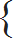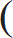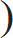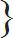# Problems on Simplification - Aptitude test, questions, shortcuts, solved example videos

Video on Simplification - shortcuts, tips and tricks

## Simplifications

Simplification is a chapter which depends on the following chapters. Hence, when dealing with simplifications u must remember all basic concepts and tricks used to solve these numerical on simplification.

1) Numbers
2) H.C.F. and L.C.M.
3) Decimal fractions
4) Square and cube roots
5) Surds and indices

Important points to Remember:

1) This chapter is very important because numerical on simplification are based on concepts related decimal fraction, square and cube roots, H.C.F. and L.C.M., numbers, etc.
2) How to find H.C.F. and L.C.M.
3) Tricks to solve decimal fractions
4) Number system, types of numbers
5) How to find square root and cube root

Basic Formulae: (Must Remember)

1) (a - b)2 = (a2 + b2 - 2ab)
2) (a + b)2 = (a2 + b2 + 2ab)
3) (a + b) (a – b) = (a2 – b2 )
4) (a3 + b3) = (a + b) (a2 – ab + b2)
5) (a3 - b3) = (a - b) (a2 – ab + b2)
6) (a + b + c)2 = a2 + b2 + c2 + 2 (ab + bc + ca)
7) (a3 + b3 + c3 – 3abc) = (a + b + c) (ar>2 + b2 + c2 – ab – bc – ac)

Quick Tips and Tricks

1) Virnaculum (Bar ‘¬¬–’): If a given expression contains a virnaculum (bar), then first simplify the given expression under virnaculum and then follow the rule of ‘BODMAS’.

Step1: When simplifying the given expressions, first brackets are to be removed in the order: ‘––’, ‘( )’, ‘{ }’, ‘[ ]’

Step2: The operations are to be performed strictly in the order: Division, Multiplication, Addition and Subtraction

2) ‘BODMAS Rule’: This rule is used to solve and find out the value of given expressions by performing the operations in a correct sequence.

BODMAS is the shortcut used to remember the procedure of simplification.

B: Bracket
O: Order (i.e. power, square root etc.)
D: Division (Left to right)
M: Multiplication (Left to right)
S: Subtraction (Left to right)

Step1: When simplifying the given expressions, first brackets are to be removed in the order: ( ), { }, [ ]

Step2: The operations are to be performed strictly in the order: Division, Multiplication, Addition and Subtraction

3) Modulus of a real number
The modulus of a real number or the absolute value is defined as follows:
Modulus of x is written as |x|
 |x| =x,          if x > 0 – x,           if x < 0
Question Variety

Type 1: Simplify

Q 1. Simplify: y – [y – (x + y) – {y –(y – x – y )} + 2x]

a. 0
b. x
c. 4x
d. x/2
View solution

Correct Option: (c)

Step 1: Solve the expression below bar
y-[y-(x+y)-{y-(y-x+y) }+2x]

Step 2: Solve () bracket
y-[y-(x+y)-{y-(2y-x) }+2x]
y-[-x-{y-2y+x}+2x]

Step 2: Solve {} bracket
y-[-x-{y-2y+x+2x}]
y-[-x-{-y+3x}]
y-[-x+y-3x]

Step 3: Solve [ ] bracket
y+4x-y
4x

Therefore, correct answer is 4x i.e option (c)

Q 2. Simplify: 13.05 × 4.5 ÷ 0.5

a. 117.45
b. 112.32
c. 115.50
d. 120.60
View solution

Correct Option: (a)

No bar, No bracket. Hence, first step starts from ‘DMAS’

Step 1: Divide 4.5 ÷ 0.5=9

Step 2: Multiply 13.05 × 9 = 117.45

 Q 3. Simplify: 10 –5 1 –7 1 + 2.8 ÷ x× 4.25 ÷ (0.2)2 = 306 2 2

a. 1.963
b. 2.847
c. 3.562
d. 4.963
View solution

Correct Option: (c)

Step 1:

 10 –5 1 –15 + 2.8 ÷ x× 4.25 ÷ (0.2)2 = 306 2 2
 10 – {5.5x – 7.5x – 2.8} × 425 = x 4

Step 2:
 10 – {–2x – 2.8} × 106.25 = 306 x
 10 –{–212.5x – 297.5x}= 306 x

Step 3:
 10x + 212.5x + 297.5 = 306 x
(306-222.5)x=297.5
 x = 297.5 = 3.562 (306 – 222.5)

Type 2: Find the value of ––––––

 Q 4. If x = 3 and 8x + 5y = 22, then find the value of x. y 4

a. 3.2
b. 2.1
c. 2.3
d. 1.5
View solution

Correct Option: (d)

 y = 4 x 3
Substitute this value in 8x+5y=22
 8x + 5 4 x = 22 3
44x=66
x=1.5

 Q 5. If 2x + 3y = 34 and x + y = 13 , then find the value of 6x + 4y. y 8

a. 62
b. 58
c. 108
d. 122
View solution

Correct Option: (a)

Step 1: Find the value of x and y

 x + y = 13 y 8
8x+8y=13y
8x-5y=0-----(1)
Multiply 2x+3y=34 by 4
8x+12y=136----(2)
Adding (1) and (2) we get,
-17y=-136
y=8
Substitute value of y = 8, in (1)
2x+3 × 8=34
x=5

Step 2: Find the value of 6x + 4y
The value of 6x+4y=(6 × 5)+(4 × 8)= 62

Type 3: Numerical on Population/Expenditure/Distribution of money/articles, etc

Q 6. The population of a city A which is 68000 decreases at the rate of 1200/year. Population of city B which is 42000, increases at the rate of 800 per year. Find in how many years, the population of cities A and B are equal?

a. 9 years
b. 10 years
c. 13 years
d. 15 years
View solution

Correct Option: (c)

We have to find the population of cities A and B after x years.

Step 1: Population of city A = 68000, decreases at the rate of 1200/year
68000 – 1200x

Step 2: Population of city B = 42000, increases at the rate of 800/year
42000 + 800x

Step 3: Find after how many population of cities A and B are equal.

Population of city A = Population of city B
68000 – 1200x = 42000 + 800x
68000 – 42000 = 1200x + 800x
26000 = 2000x
x = 13

Q 7. A contractor pays Rs. 20 to a worker for each day and the worker forfeits Rs. 10 for each day if he is idle. At the end of 60 days, the worker gets Rs. 300. Find for how many days the worker was idle?

a. 28 days
b. 30 days
c. 34 days
d. 40 days
View solution

Correct Option: (b)

Step 1: Number of days worked by the worker = 60 and he remained idle for x days. Therefore, number of days worked = (60 – x)

Step 2: Each day he was getting paid Rs. 20. Therefore, the payment received for working days = (60 – x) 20

Step 3: After subtracting the amount which he forfeited, he receives Rs. 300.

Therefore,
(60 – x) 20 – 10x = 300
1200 – 20x – 10x = 300
900 = 30x
x = 30 days

Q 8. In a farm, along with 50 hens, there were 45 goats and 8 horses and some farmers. If total number of feet be 224 more than number of heads, then find the number of farmers.

a. 11
b. 15
c. 16
d. 18
View solution

Correct Option: (b)

Let’s the number of farmers be y.

Step 1: Find number of heads
= (50 hens + 45 goats + 8 horses + y farmers)
= (103 + y)

Step 2: Number of feet
= [(Hens 2 × 50) + (45 × 4) + (8 × 4) + (y × 2)]
= [100 + 180 + 32 + 2y]
= 312 + 2y

Step 3: Find number of farmers
(312 + 2y) – (103 + y) = 224
312 + 2y – 103 – y = 224
y = 15

Number of farmers = 15

Square Root and Cube Root - Aptitude test, questions, shortcuts, solved example videos
Square root & Cube root - Quantitative aptitude tutorial with easy tricks, tips & short cuts explaining the concepts. Online aptitude preparation material with practice question bank, examples, solutions and explanations. Video lectures to prepare quantitative aptitude for placement tests & competitive exams like MBA, Bank exams, RBI, IBPS, SSC, SBI, RRB, Railway, LIC, MAT. Very useful for freshers, engineers & software developers taking entrance exams. Learn and take practice tests!
Surds and Indices - Aptitude test, questions, shortcuts, solved example videos
Surds and Indices - Quantitative aptitude tutorial with easy tricks, tips & short cuts explaining the concepts. Online aptitude preparation material with practice question bank, examples, solutions and explanations. Video lectures to prepare quantitative aptitude for placement tests & competitive exams like MBA, Bank exams, RBI, IBPS, SSC, SBI, RRB, Railway, LIC, MAT. Very useful for freshers, engineers & software developers taking entrance exams. Learn and take practice tests!
Ratio and Proportion - Aptitude test, questions, shortcuts, solved example videos
Ratio and Proportion - Quantitative aptitude tutorial with easy tricks, tips, short cuts explaining the concepts. Online aptitude preparation material with practice question bank, examples, solutions and explanations. Video lectures to prepare quantitative aptitude for placement tests, competitive exams like MBA, Bank exams, RBI, IBPS, SSC, SBI, RRB, Railway, LIC, MAT. Very useful for freshers, engineers, software developers taking entrance exams. Learn and take practice tests!